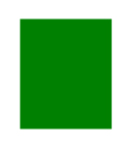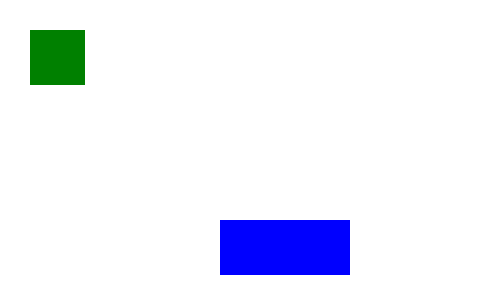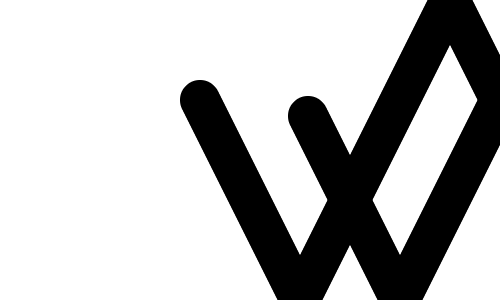# HTML5 Canvas Tag Intro

This is a walk through/tutorial on the basics of the HTML5 Canvas Tag. We will start by creating some simple shapes and then move on to some animation. To download the code and follow along you can check out the github repo here.

###Canvas Setup Start with a basic HTML5 document, in the body add your <canvas> tag. The tag will need at least three things; an id, a width, and a height. Like this:

``````<canvas id="myCanvas" width="500" height="300">
Your browser does not support this.
</canvas>``````

The id is needed for JavaScript to be able to interact with the canvas. The width and height are used to give the canvas dimensions, although there is a default size of 300 by 150 pixels. You do not need to add “px” to the end of the dimension attributes, they already represent the size in pixels. It’s best to use these attributes instead of using css styles as css tends to take the default canvas size and stretch it causing the canvas drawings to look out of shape. When I tried using css to size my canvas it stretched a square of 50px by 50px to look like a big rectangle.

The Square before css styling:The same square after the canvas was resized with css:Anything that you put between the opening and closing canvas tags will not be displayed, unless the browser does not support HTML5 canvas tags. You could add links to supported browsers here for users to get.

At the end of the html body you must include your JavaScript file where we will interact with the canvas.

``<script src="js/basicShapes.js"></script>``

###Working With Canvas

To get started with interacting with your canvas you’ll need a function with two things.

First declare your function {% highlight javascript %} function draw() {

} {% endhighlight %}

Next you will store your canvas in a variable by selecting the canvas element by the Id you gave earlier: {% highlight javascript %} var canvas = document.getElementById(‘myCanvas’); {% endhighlight %}

And then you need to set the canvas context to enable 2d drawings: {% highlight javascript %} var context = canvas.getContext(‘2d’); {% endhighlight %}

Then simply call your draw function when the window is loaded: {% highlight javascript %} window.onload = draw; {% endhighlight %}

The canvas can now be used for drawing and animating shapes and objects.

###Drawing Simple Rectangles Now that we have the canvas context set we can use it to draw shapes. Drawing shapes is done in the same draw() function we declared above.

To draw a small green square we need to do two things. First use fillStyle to set the colour: {% highlight javascript %} context.fillStyle = ‘green’; {% endhighlight %}

Then we use fillRect to set the position and the size of the rectangle: {% highlight javascript %} context.fillRect(30, 30, 55, 55); {% endhighlight %} This creates the same green square that was shown above.

The fillRect method does two things and can be split up into two separate methods, fill and rect.

• The rect method takes four parameters to make the rectangle. The first two are the X and Y coordinates where the rectangle will start on the canvas. The last two parameters are for the WIDTH and HEIGHT of the rectangle.

• The fill method is used to actually draw the rectangle on the canvas. This doesn’t need any parameters

Here’s another rectangle example: {% highlight javascript %} context.fillStyle = ‘blue’; context.fillRect(220, 220, 130, 55); {% endhighlight %}###Drawing basic lines

Drawing lines is similar to rectangles but they take a little bit more to draw.

• The line path first needs to be started {% highlight javascript %} context.beginPath(); {% endhighlight %}

• Then set the coordinate for the beginning of the line {% highlight javascript %} context.moveTo(100, 150); {% endhighlight %}

• Set the end coordinate of the line {% highlight javascript %} context.lineTo(450, 50); {% endhighlight %}

• (optional) Set the line width in pixels, default 1px {% highlight javascript %} context.lineWidth = 10; {% endhighlight %}

• (optional) Set the line colour with a strokeStyle, default is black {% highlight javascript %} context.strokeStyle = ‘red’; {% endhighlight %}

• (optional) Change the look of the end of the line. Adding a lineCap of ‘round’ will add a rounded point to the line {% highlight javascript %} context.lineCap = ‘round’; {% endhighlight %}

• Then finally to draw the line with stroke {% highlight javascript %} context.stroke(); {% endhighlight %} This makes a straight red line:Lines can have multiple points and angle changes. By adding more lineTo methods a line could look like this: {% highlight javascript %} context.beginPath(); context.moveTo(50, 290); context.lineTo(140, 200); context.lineTo(400, 100); context.lineTo(400, 10); context.lineTo(250, 10); context.lineWidth = 5; context.strokeStyle = ‘orange’; context.stroke(); {% endhighlight %}###Bouncing Ball Animation

This demo makes a small ball bounce around the walls of the canvas. Every 20 milliseconds the the canvas gets cleared and the ball is redrawn in a new position, making it look like it is moving. When the ball gets to one of the walls of the canvas it changes direction looking like it bounced off.

Here’s what we will be making (actual canvas, not just a gif):

Your browser does not support this.

To start we’ll set some global variables {% highlight javascript %} var canvas; var context; var x = 200; //start position var y = 100; //start position var mx = 2; //x coordinate translation var my = 4; //y coordinate translation var WIDTH = 500; //canvas boundaries var HEIGHT = 300; //canvas boundaries {% endhighlight %}

Next we will create a init function to set the canvas and the animation interval {% highlight javascript %} function init() { canvas = document.getElementById(“myCanvas”); context = canvas.getContext(“2d”); return setInterval(draw, 20); } {% endhighlight %} The canvas and the context are set here just like in the first demo. The third line sets the interval where every 20 milliseconds a method called draw will be called.

Before we create the draw method we will first make a method that draws circles {% highlight javascript %} function circle(x,y,r) { context.beginPath(); context.arc(x,y,r,0, Math.PI*2, true); context.fill(); } {% endhighlight %}

• The circle method takes three parameters; an X coordinate, a Y coordinate, and a radius or the size of the circle.
• We first initiate the circle by calling the beginPath method.
• Next we use the arc method to construct the circle. We give arc the X and Y coordinates and the Radius, the next parameter is the start angle of the circle, then we use “Math.Pi*2” to create the end angle which makes the arc a full circle (This could be changed to make ovals or semi circles), and the last parameter is called anticlockwise which just draws the arcs angle in a given direction.

Now we can start building the draw function {% highlight javascript %} function draw() { circle(x, y, 20); } {% endhighlight %} Every time the draw function is called it will call the circle function with the X and Y coordinates that we set at the beginning, and a radius of 20. So far this will only draw a static circle. {% highlight javascript %} //Check to see if the ball is a side of the canvas if (x + mx > WIDTH || x + mx < 0) { mx = -mx; //change the x direction } //checkif the ball is at the top or bottom of the canvas if (y + my > HEIGHT || y + my < 0) { my = -my; //change the y direction } x += mx; //change the next x coordinate of the ball y += my; //change the next y coordinate of the ball {% endhighlight %} * The first if statement checks to see if the next position of the ball will hit one of the sides of the canvas. If it will then the X movement of the ball gets reversed, this makes the ball “bounce” off the wall. * The second if statement does the same thing but for the top and bottom of the canvas. If the ball gets to one of these edges it will reverse it’s Y movement. * The last two lines changes the position variables making the X and Y coordinates for positioning the circle different every time the draw method is called. * With this the circle will move and bounce around the canvas like it should. However, it will leave behind a trail of every circle that gets drawn, like this:The last thing we need to do is clear the canvas of the old circles so they aren’t left behind in a big line behind the bouncing ball. We will make a method to clear the canvas {% highlight javascript %} function clear() { //remove any drawings from the canvas context.clearRect(0,0, WIDTH, HEIGHT); } {% endhighlight %} * In the clear function we call the clearRect on the canvas context. This makes a rectangle, similar to what we made in the first demo, and clears everything inside of it. The clearRect method takes in four parameters; the X and Y coordinates of the top left corner of the rectangle, and the Width and Height of the rectangle. Anything within the rectangle gets cleared or erased.

Next we need to call the clear function before the circle is drawn in the draw method. {% highlight javascript %} function draw() { clear(); //call the clear function … … } {% endhighlight %} Now the ball will bounce around the canvas without leaving a trail behind it.

###References Basic HTML5 Canvas Tutorials:

http://www.html5canvastutorials.com/tutorials/html5-canvas-rectangles/

HTML5 Canvas Tag Tutorial Learn to Draw and Animate Using Javascript: